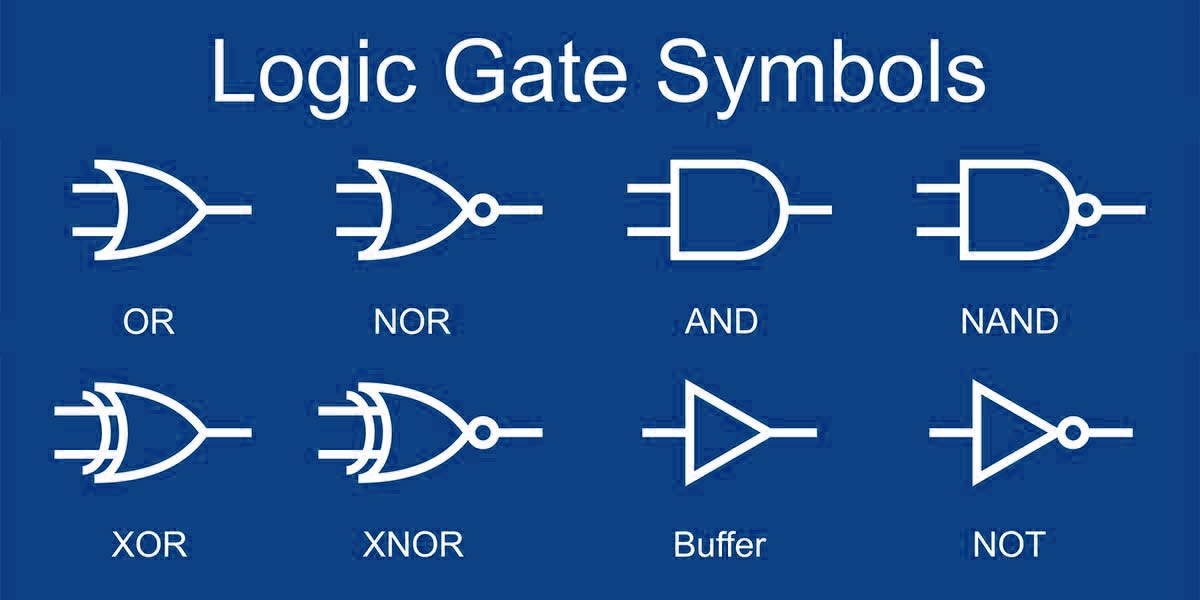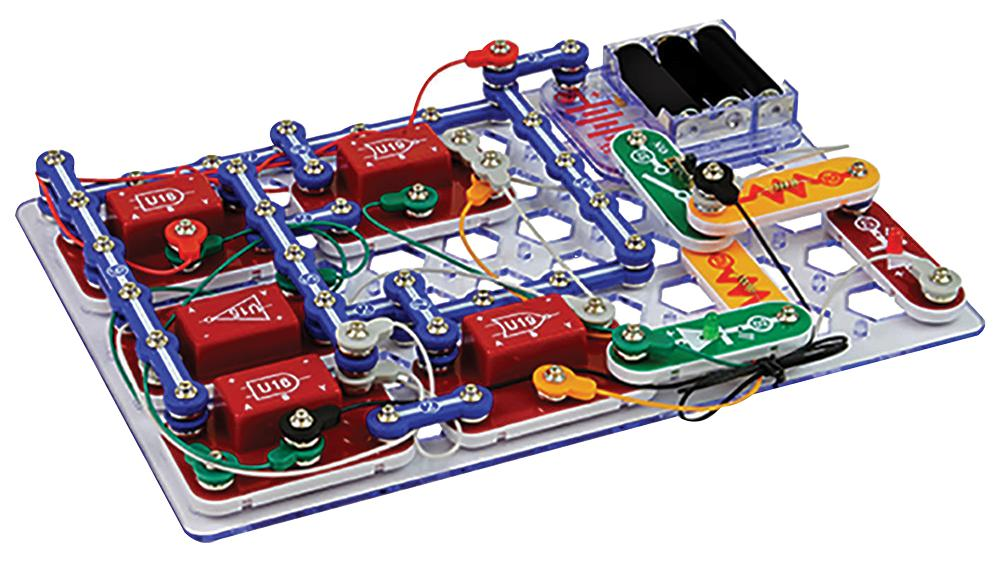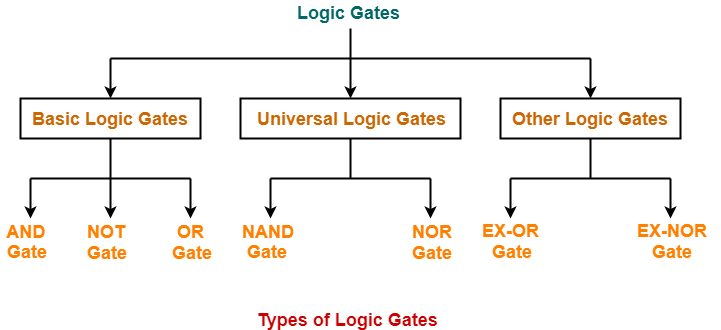# Digital Circuits Logic Gates Examples

By | March 19, 2023

Logic gates are the building blocks of digital circuits, providing powerful and versatile solutions to complex problems. A logic gate is an electronic component that takes one or more input signals and produces a single output signal based on a certain logical operation. Each logic gate has a different function, and when these basic elements are combined, they enable complex computational tasks to be performed.

Understanding the principles behind digital circuits and logic gates is essential for anyone wanting to design and build modern electronic devices. In this article, we'll explore the basics of logic gates and provide some examples of how they can be used in real-world applications.

At its most basic level, a logic gate is a device that takes two values - 1 and 0 - as inputs and compares them to produce an output of either 1 or 0. These 1s and 0s can represent any kind of binary data, such as true/false, on/off, yes/no, etc. The output signal will be determined by the type of logic gate being used.

The three most common logic gates are the AND, OR, and NOT gates. The AND gate takes two inputs and produces an output of 1 only if both inputs are 1. Conversely, the OR gate produces an output of 1 if either or both of its inputs are 1. Finally, the NOT gate takes a single input and produces an output of 0 if the input is 1 and 1 if the input is 0.

Logic gates can be combined in various ways to create more complex logic circuits. For example, the NAND gate is a combination of an AND gate and a NOT gate, and it produces an output of 0 if both inputs are 1. Similarly, the NOR gate is a combination of an OR gate and a NOT gate, and it produces an output of 0 if either input is 1.

Real-world examples of logic gates can be found almost everywhere. Your computer's central processing unit (CPU) contains thousands of logic gates, allowing the processor to perform sophisticated calculations. Logic gates are also used to control airbag systems in cars, automated door locks, and even some toys.

In conclusion, logic gates are an essential component of digital circuits, providing powerful and versatile solutions to complex problems. By combining different types of logic gates, complex computational tasks can be performed. Examples of logic gates can be found in everyday life, from your computer's CPU to your car's airbag system. Understanding their principles and applications is essential for anyone wanting to build modern electronic devices.Small Logic Gates The Building Blocks Of Digital Circuits Part 2 Nuts Volts MagazineDigital Logic Sparkfun LearnLogic Gate An Overview Sciencedirect TopicsSolved Electrical Logic Circuit Help Autodesk CommunitySamplelogic4 GifThe Basic Elements Of Digital Circuits Mosfet Gate And LogicScdlg200 Snap Circuits Digital Logic Gate Project Kit CpcAlternative Logic Gates Bubbled Gate VidyalayWhat Are Basic Logic GatesLogicly A Logic Circuit Simulator For Windowacos Gates Flip Flops Computer Architecture Electronics Integrated CircuitsCircuit Simplification Examples Boolean Algebra Electronics TextbookDigital Electronics And Logic Circuits Role Of TransistorsLogic Gates With Microcontroller EewebDigital Electronics Logic Gates Basics Tutorial Circuit Symbols Truth TablesDigital Circuits And Systems I Sistemes Digitals Csd Eetac UpcBasic Logic Gates Types Functions Truth Table Boolean ExpressionsHow To Use Digital Logic In Electronic Circuits Circuit BasicsDigital Logic Circuits Defination Application And TypesLogic Gates Software Draw Online Creately